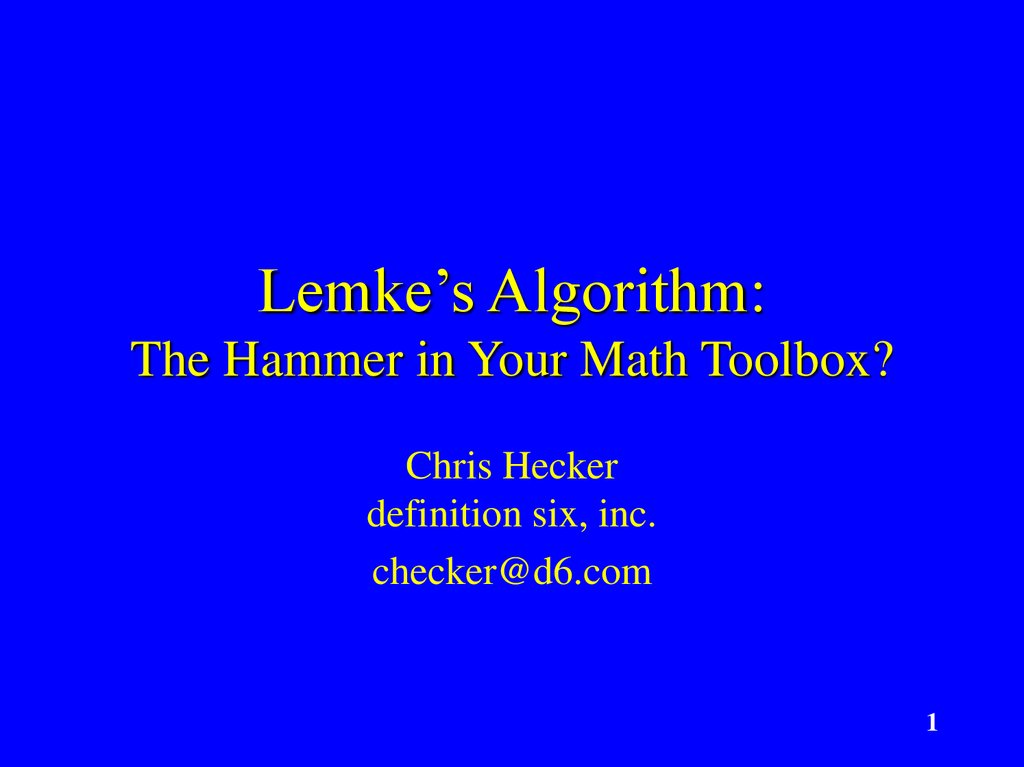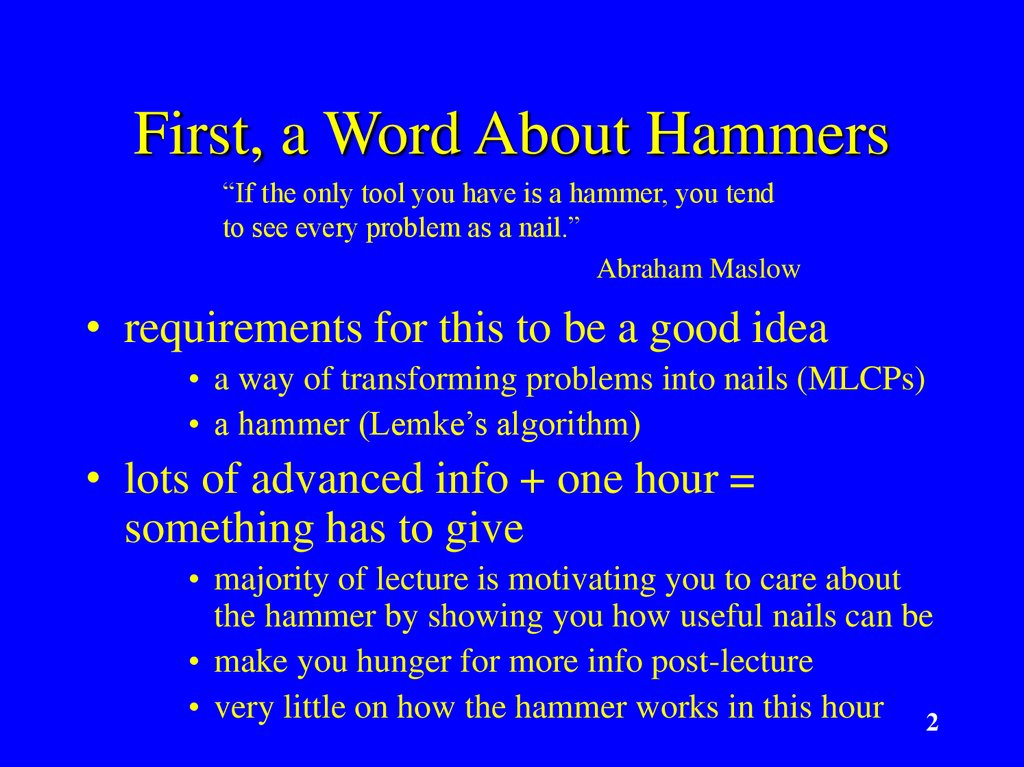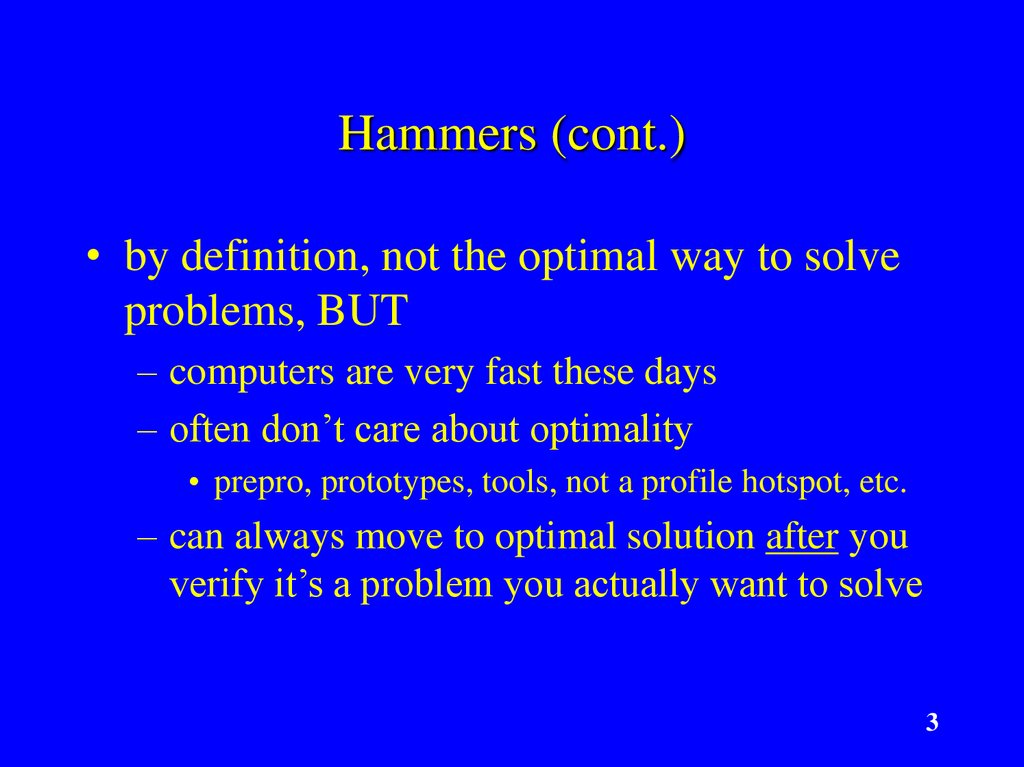# Lemke’s Algorithm: The Hammer in Your Math Toolbox?

## 1. Lemke’s Algorithm: The Hammer in Your Math Toolbox?

Chris Hecker
definition six, inc.
[email protected]
1

## 2. First, a Word About Hammers

“If the only tool you have is a hammer, you tend
to see every problem as a nail.”
Abraham Maslow
• requirements for this to be a good idea
• a way of transforming problems into nails (MLCPs)
• a hammer (Lemke’s algorithm)
• lots of advanced info + one hour =
something has to give
• majority of lecture is motivating you to care about
the hammer by showing you how useful nails can be
• very little on how the hammer works in this hour 2

## 3. Hammers (cont.)

• by definition, not the optimal way to solve
problems, BUT
– computers are very fast these days
– often don’t care about optimality
• prepro, prototypes, tools, not a profile hotspot, etc.
– can always move to optimal solution after you
verify it’s a problem you actually want to solve
3

## 4. What are “advanced game math problems”?

• problems that are ammenable to
mathematical modeling
• state the problem clearly
• state the desired solution clearly
• describe the problem with equations so a proposed
solution’s quality is measurable
• figure out how to solve the equations
• why not hack it?
• I believe better modeling is the future of game
technology development (consistency, not reality)
4

## 5. Prerequisites

• linear algebra
• vector, matrix symbol manipulation at least
• calculus concepts
• what derivatives mean
• comfortable with math notation and
concepts
5

## 6. Overview of Lecture

• random assortment of example problems
breifly mentioned
• 5 specific example problems in some depth
• including one that I ran into recently and how I
solved it
• generalize the example models
• transform them all to MLCPs
• solve MLCPs with Lemke’s algorithm
6

## 7. A Look Forward

• linear equations
Ax = b
min ½ xTQx + cTx
s.t. Ax >= b
• linear inequalities
Dx = e
Ax >= b
• linear programming • linear complimentarity problem
a = Af + b
min cTx
a >= 0, f >= 0
s.t. Ax >= b, etc.
aifi = 0
7

## 8. Applications to Games graphics, physics, ai, even ui

computational geometry
visibility
contact
curve fitting
constraints
integration
graph theory
network flow
economics
site allocation
game theory
IK
machine learning
image processing
8

## 9. Applications to Games (cont.)

• don’t forget...
– The Elastohydrodynamic Lubrication Problem
– Solving Optimal Ownership Structures
• “The two parties establish a relationship in which
they exchange feed ingredients, q, and manure, m.”
9

## 10. Specific Examples #1a: Ease Cubic Fitting

• warm up with an ease
curve cubic
x(t)=at3+bt2+ct+d
x’(t)=3at2+2bt+c
• 4 unknowns a,b,c,d
(DOFs) we get to set, we
choose:
x(0) = 0, x(1) = 1
x’(0) = 0, x’(1) = 0
1
x
0
0
t
1
10

## 11. Specific Examples #1a: Ease Cubic Fitting (cont.)

• x(t)=at3+bt2+ct+d,
x(0) = a03+b02+c0+d
x(1) = a13+b12+c1+d
x’(0) = 3a02+2b0+c
x’(1) = 3a12+2b1+c
x’(t)=3at2+2bt+c
=d=0
= a+b+c+d = 1
=c=0
= 3a + 2b + c = 0
11

## 12. Specific Examples #1a: Ease Cubic Fitting (cont.)

• d = 0, a+b+c+d = 1, c = 0, 3a + 2b + c = 0
• a+b=1, 3a+2b=0
• a=1-b => 3(1-b)+2b = 3-3b+2b = 3-b = 0
• b=3, a=-2
• x(t) = 3t2 - 2t3
12

## 13. Specific Examples #1a: Ease Cubic Fitting (cont.)

• or,
x(0) =
d
x(1) = a + b + c + d
x’(0) =
c
x’(1) = 3a + 2b + c
x(0)
0 0
x(1)
1 1
=
x’(0) 0 0
x’(1) 3 2
0
1
1
1
1
1
0
0
=0
=1
=0
=0
a
b
=
c
d
0
1
0
0
(can solve for any rhs)
Ax = b, a system of linear equations
13

## 14. Specific Examples #1b: Cubic Spline Fitting

• same technique to fit
higher order polynomials,
but they “wiggle”
• piecewise cubic is better
“natural cubic spline”
• xi(ti)=xi
xi(ti+1)=xi+1
x’i(ti) - x’i-1(ti) = 0
x’’i(ti) - x’’i-1(ti) = 0
• there is coupling between
the splines, must solve
simultaneously
x2
x3
x0
t0
x1
t1
t2
t3
• 4 DOF per spline
– 2 endpoint eqns per spline
– 4 derivative eqns for inside
points
– 2 missing eqns = endpoint
slopes
14

## 15. Specific Examples #1b: Cubic Spline Fitting (cont.)

xi(ti)=xi
xi(ti+1)=xi+1
x’i(ti) - x’i-1(ti) = 0
x’’i(ti) - x’’i-1(ti) = 0
..
.
a0
b0
c0
d0
a1
b1
c1
d1
..
.
=
x0
x1
0
Ax = b, a
0
system of
x1
x2 linear equations
0
0
..
.
15

## 16. Specific Examples #2: Minimum Cost Network Flow

• what is the cheapest flow
route(s) from sources to sinks?
• model, want to minimize cost
cij = cost of i to j arc
bi = i’s supply/demand, sum(bi)=0
xij = quantity shipped on i to j arc
x*k = sum(xik) = flow into k
xk* = sum(xki) = flow out of k
• flow balance: x*k - xk* = -bk
• one-way streets: xij >= 0
16

## 17. Specific Examples #2: Minimum Cost Network Flow (cont.)

• min cost: minimize cTx
• the sum of the costs times the
quantities shipped (cTx = c ·x)
• flow balance is coupling: matrix
x*k - xk* = -bk
xac
-1 -1 -1 1 0 0 0 0 1 0…
xae
0 0 0 -1 -1 -1 1 …
xba
...
xbc
xbe
xdb
..
.
ba
bb
= - bc
bd
.
.
.
minimize cTx
subject to
Ax = -b
x >= 0
a linear
programming
17
problem

## 18. Specific Examples #3: Points in Polys

• point in convex poly
defined by planes
n1 · x >= d1
n2 · x >= d2
n3 · x >= d3
Ax >= b,
linear inequality
n3
n2
x
n1
• farthest point in a
direction in poly, c:
min -cTx
s.t. Ax >= b
linear programming
18

## 19. Specific Examples #3: Points in Polys (cont.)

• closest point in two polys
min (x2-x1)2
s.t. A1x1 >= b1
A2x2 >= b2
• stack ‘em in blocks, Ax >= b
x1
x= x
2
b1
b= b
2
A = A1 A2
n3
n2
x1
n1
x2
what about (x2-x1)2, how do we stack it?
19

## 20. Specific Examples #3: Points in Polys (cont.)

• how do we stack x1,x2 into single x given
(x2-x1)2 = x22-2x2•x1+x12
x1T x2T
min xTQx
s.t. Ax >= b
1 -1
-1 1
x1
2-2x • x +x 2 = xTQx
=
x
2
2
1
1
x2
x2 = xTx = x · x
1 = identity matrix
20

## 21. Specific Examples #3: Points in Polys (cont.)

• more points, more polys!
min (x2-x1)2 + (x3-x2)2 + (x3-x1)2
x1T x2T x3T
2 -1 -1 x1
-1 2 -1 x2 = xTQx
-1 -1 2 x3
min xTQx
s.t. Ax >= b
• same form for all these poly problems
• never specified 2d, 3d, 4d, nd!
21

## 22. Specific Examples #4: Contact

• model like IK constraints
a = Af + b
a >= 0, no penetrating
f >= 0, no pulling
aifi = 0, complementarity
(can’t push if leaving)
linear complementarity problem
a1
a2
f1
a1
f2
a2
f1
f2
it’s a mixed LCP if some ai = 0, fi free,
like for equality constraints
22

## 23. Specific Examples #5: Joint Limits in CCD IK

a1
• how to do child-child constraints in CCD?
g1
• parent-child are easy, but need a way to couple
two children to limit them relative to each other
how to model this & handle all the cases?
define dn= gn - an
min (x1 - d1)2 + (x2 - d2)2
s.t. c1min <= a1+x1 - a2-x2 <= c1max
parent-child are easy in this framework:
c2min <= a1+x1 <= c2max
min xTQx
s.t. Ax >= b
a2
a1
g2
g1
23

## 24. What Unifies These Examples?

• linear equations
Ax = b
min ½ xTQx + cTx
s.t. Ax >= b
• linear inequalities
Dx = e
Ax >= b
• linear programming • linear complimentarity problem
a = Af + b
min cTx
a >= 0, f >= 0
s.t. Ax >= b, etc.
aifi = 0
24

## 25. QP is a Superset of Most

programming
min ½xTQx + cTx
s.t. Ax >= b
Dx = e
• linear equations
• Ax = b
• Q, c, A, b = 0
• linear inequalities
• Ax >= b
• Q, c, D, e = 0
• linear programming
but MLCP is a superset
of convex QP!
• min cTx
s.t. Ax >= b, etc.
• Q, etc. = 0
25

## 26. Karush-Kuhn-Tucker Optimality Conditions get us to MLCP

• for QP
• form “Lagrangian”
min ½ xTQx + cTx
s.t. Ax - b >= 0
Dx - e = 0
L(x,u,v) = ½ xTQx + cTx - uT(Ax - b) - vT(Dx - e)
• for optimality (if convex):
L/ x = 0
Ax - b >= 0
Dx - e = 0
u >= 0 ui(Ax-b)i = 0
– this is related to basic calculus min/max f’(x) = 0 solve
26

## 27. Karush-Kuhn-Tucker Optimality Conditions (cont.)

• L(x,u,v) = ½ xTQx + cTx - uT(Ax - b) - vT(Dx - e)
• y = L/ x = Qx + c - ATu - DTv = 0, x free
• w = Ax - b >= 0, u >= 0, wiui = 0
• s = Dx - e = 0, v free
y
Q -DT -AT
s = D 0 0
w
A 0 0
x
c
v + -e
u
-b
y, s = 0
x, v free
w, u >= 0
wiui = 0
27

## 28. This is an MLCP

y
Q -DT -AT
s = D 0 0
w
A 0 0
x
c
v + -e
u
-b
a
f
=
aifi = 0
A
+
y, s = 0
x, v free
w, u >= 0
wiui = 0
b
some a >= 0, some = 0
some f >= 0, some free
(but they correspond so complementarity holds)
28

## 29. Modeling Summary

• a lot of interesting problems can be
formulated as MLCPs
– model the problem mathematically
– transform it to an MLCP
– on paper or in code with wrappers
– but what about solving MLCPs?
29

## 30. Solving MLCPs (where I hope I made you hungry enough for homework)

• Lemke’s Algorithm is only about 2x as
complicated as Gaussian Elimination
• Lemke will solve LCPs, which some of
these problems transform into
• then, doing an “advanced start” to handle
the free variables gives you an MLCP
solver, which is just a bit more code over
plain Lemke’s Algorithm
30

## 31. Playing Around With MLCPs

• PATH, a MCP solver (superset of MLCP!)
• really stoked professional solver
• free version for “small” problems
• matlab or C
• OMatrix (Matlab clone) free trial (omatrix.com)
• only LCPs, but Lemke source is in trial
» not a great version, but it’s really small (two pages of code)
and quite useful for learning, with debug output
» good place to test out “advanced starts”
• my Lemke’s + advanced start code
• not great, but I’m happy to share it
• it’s in Objective Caml :)
31

## 32. References for Lemke, etc.

free pdf book by Katta Murty on LCPs, etc.
free pdf book by Vanderbei on LPs
The LCP, Cottle, Pang, Stone
Practical Optimization, Fletcher
web has tons of material, papers, complete books,
etc.
• email to authors
• relatively new math means authors are still alive, bonus!
32

33

## 34. Specific Examples #5: Constraints for IK

• compute “forces” to keep bones together
a1 = A11 f1 + b1
f1
fe
a1 : relative acceleration
at constraint
f1 : force at constraint
b1 : external forces converted to
accelerations at constraints
A11 : force/acceleration relation matrix
34

## 35. Specific Examples #5: Constraints for IK (cont.)

• multiple bodies gives coupling...
a1 A11 A12 f1
b1
a2 = A21 A22 f2 + b2
a = Af + b
a = 0 for rigid constraints
Af = -b, linear equations
fe
f1
f2
35